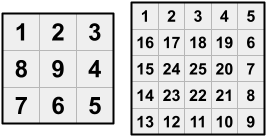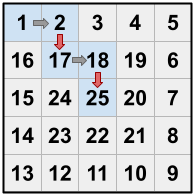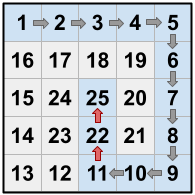시간 제한메모리 제한제출정답맞힌 사람정답 비율
서브태스크 참고 (추가 시간 없음) 1024 MB43393790.244%

## 문제

As punishment for being naughty, Dante has been trapped in a strange house with many rooms. The house is an $\mathbf{N} \times \mathbf{N}$ grid of rooms, with $\mathbf{N}$ odd and greater than $1$. The upper left room is numbered $1$, and then the other rooms are numbered $2$, $3$, ..., $\mathbf{N}^2$, in a clockwise spiral pattern. That is, the numbering proceeds along the top row of the grid and then makes a 90 degree turn to the right whenever a grid boundary or an already numbered room is encountered, and finishes in the central room of the grid. Because $\mathbf{N}$ is odd, there is always a room in the exact center of the house, and it is always numbered $\mathbf{N}^2$.

For example, here are the room numberings for houses with $\mathbf{N} = 3$ and $\mathbf{N} = 5$:Dante starts off in room $1$ and is trying to reach the central room (room $\mathbf{N}^2$). Throughout his journey, he can only make moves from his current room to higher-numbered, adjacent rooms. (Two rooms must share an edge — not just a corner — to be adjacent.)

Dante knows that he could walk from room to room in consecutive numerical order — i.e., if he is currently in room $x$, he would move to room $x+1$, and so on. This would take him exactly $\mathbf{N}^2 - 1$ moves. But Dante wants to do things his way! Specifically, he wants to reach the central room in exactly $\mathbf{K}$ moves, for some $\mathbf{K}$ strictly less than $\mathbf{N}^2 - 1$.

Dante can accomplish this by taking one or more shortcuts. A shortcut is a move between rooms that are not consecutively numbered.

For example, in the $5 \times 5$ house above,

• If Dante is at $1$, he cannot move to $17$, but he can move to $2$ or to $16$. The move to $2$ is not a shortcut, since $1 + 1 = 2$. The move to $16$ is a shortcut, since $1 + 1 \neq 16$.
• From $2$, it is possible to move to $3$ (not a shortcut) or to $17$ (a shortcut), but not to $1$, $16$, or $18$.
• From $24$, Dante can only move to $25$ (not a shortcut).
• It is not possible to move out of room $25$.

As a specific example using the $5 \times 5$ house above, suppose that $\mathbf{K}$ = $4$. One option is for Dante to move from $1$ to $2$, then move from $2$ to $17$ (which is a shortcut), then move from $17$ to $18$, then move from $18$ to $25$ (which is another shortcut). This is illustrated below (the red arrows represent shortcuts):Can you help Dante find a sequence of exactly $\mathbf{K}$ moves that gets him to the central room, or tell him that it is impossible?

## 입력

The first line of the input gives the number of test cases, $\mathbf{T}$. $\mathbf{T}$ test cases follow. Each test case consists of one line with two integers $\mathbf{N}$ and $\mathbf{K}$, where $\mathbf{N}$ is the dimension of the house (i.e. the number of rows of rooms, which is the same as the number of columns of rooms), and $\mathbf{K}$ is the exact number of moves that Dante wants to make while traveling from room $1$ to room $\mathbf{N}^2$.

## 출력

For each test case, output one line containing Case #x: y, where $x$ is the test case number (starting from 1).

If no valid sequence of exactly $\mathbf{K}$ moves will get Dante to the central room, $y$ must be IMPOSSIBLE.

Otherwise, $y$ must be an integer: the number of times that Dante takes a shortcut, as described above. (Notice that because Dante wants to finish in strictly less than $\mathbf{N}^2 - 1$ moves, he must always use at least one shortcut.) Then, output $y$ more lines of two integers each. The $i$-th of these lines represents the $i$-th time in Dante's journey that he takes a shortcut, i.e., he moves from some room $a_i$ to another room $b_i$ such that $a_i + 1 \lt b_i$.

Notice that because these lines follow the order of the journey, $a_i \lt a_{i+1}$ for all $1 \le i \lt y$.

## 제한

• $1 \le \mathbf{T} \le 100$.
• $1 \le \mathbf{K} \lt \mathbf{N}^2 - 1$.
• $\mathbf{N} \mod 2 \equiv 1$. ($\mathbf{N}$ is odd.)

## Test Set 1 (3점)

시간 제한: 5 초

• $3 \le \mathbf{N} \le 9$.

## Test Set 2 (4점)

시간 제한: 20 초

• $3 \le \mathbf{N} \le 39$.

## Test Set 3 (13점)

시간 제한: 20 초

• $3 \le \mathbf{N} \le 9999$.

## 예제 입력 1

4
5 4
5 3
5 12
3 1


## 예제 출력 1

Case #1: 2
2 17
18 25
Case #2: IMPOSSIBLE
Case #3: 2
11 22
22 25
Case #4: IMPOSSIBLE


## 힌트

Sample Case #1 is described in the problem statement. Dante's route is $1 \to 2 \to 17 \to 18 \to 25$. Because $1 \to 2$ and $17 \to 18$ are moves between consecutively numbered rooms, they are not included in the output. Only the shortcuts ($2 \to 17$ and $18 \to 25$) are included.

In Sample Case #2, there is no solution. (Recall that there is no way for Dante to move diagonally.)

In Sample Case #3, observe that $22$ appears both as the end of one shortcut and the start of the next. It would not be valid to include the line 11 22 25 in the output; each line must represent a single shortcut.There is another solution that uses only one shortcut: Dante can move from $1 \to 2 \to 3 \to 4 \to 5 \to 6$, then move from $6 \to 19$ (a shortcut), then move from $19 \to 20 \to 21 \to 22 \to 23 \to 24 \to 25$. This is also valid; there is no requirement to minimize (or maximize) the number of shortcuts taken.

In Sample Case #4, Dante cannot get to the central room ($9$, in this case) in just one move.

## 채점 및 기타 정보

• 예제는 채점하지 않는다.